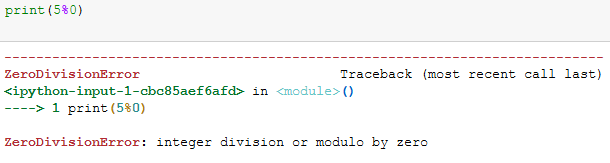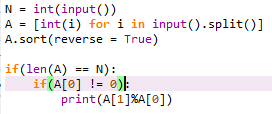# When I run my code on codechef online compiler it gives correct output for all input. but when I ran it on contest it gave rintime error

This post was flagged by the community and is temporarily hidden.

is it possible that the value of C == 0 ? if yes then you are getting run-time error due to this division.
As you can see in the snippet below also you will get a runtime error if you try to divide by 0./**** Now it give compilation error ****/

N = int(input())
A = [int(i) for i in input().split()]
A.sort(reverse = True)
if(len(A) == N):
if(A == 0):
pass
print(A%A)

it is hard to understand your code without formatting, especially python code, aw try this its running fine on my machine